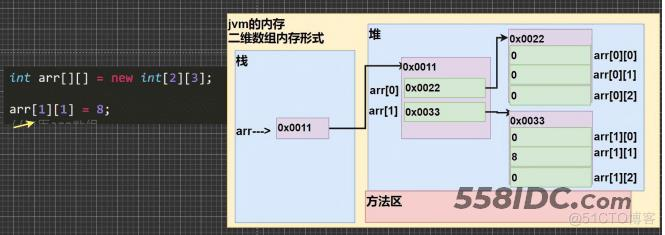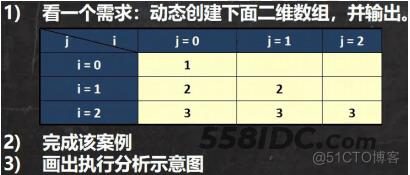﻿ java二维数组基础知识详解 - 自由资讯

# java二维数组基础知识详解

##### 目录
• 1. 查找
• 2. 顺序查找
• 3. 二分查找
• 4. 多维数组
• 4.1 二维数组 175
• 4.2 二维数组细节
• 5. 二维数组的使用方式 176
• 6. 二维数组的动态初始化
• 1.先声明：类型 数组名[][]; 再定义(开辟空间) 数组名 = new 类型[大小][大小]
• 2.动态初始化-列数不确定 178
• 7. 二维数组的静态初始化 179
• 8. 二维数组练习 180
• 8.1 int arr[][]={{4,6},{1,4,5,7},{-2}}; 遍历该二维数组，并得到和

## 1. 查找

1) 顺序查找 SeqSearch.java

2) 二分查找【二分法，放在算法讲解】

## 2. 顺序查找

有一个数列：白眉鹰王、金毛狮王、紫衫龙王、青翼蝠王猜数游戏：从键盘中任意输入一个名称，判断数列中是否 包含此名称【顺序查找】 要求: 如果找到了，就提示找到，并给出下标值。

```import java.util.Scanner;
public class Test21
{
public static void main(String[] args)
{
//有一个数列：白眉鹰王、金毛狮王、紫衫龙王、青翼蝠王猜数游戏：
//从键盘中任意输入一个名称，判断数列中是否 包含此名称【顺序查找】
// 要求: 如果找到了，就提示找到，并给出下标值。
// 思路分析 1. 定义一个字符串数组
// 2. 接收用户输入, 遍历数组，逐一比较，如果有，则提示信息，并退出
//定义一个字符串数组
Scanner myScanner = new Scanner(System.in);
String[] names = {"白眉鹰王", "金毛狮王", "紫衫龙王", "青翼蝠王"};
System.out.println("请输入名字");
String findname = myScanner.next();
//遍历数字组
int index = -1;//判断找没找到
for(int i=0;i<names.length;i++)
{
if(findname.equals(names[i]))//使用equals比较字符串是相等
{
System.out.println("恭喜你找到了"+findname);
System.out.println("下标是"+i);
index = i;
break;
}
}
if(index==-1)//没找到
{
System.out.println("没找到"+findname);
}
}
}```

## 3. 二分查找

仅当了解，后面会详细讲解

```import java.util.Scanner;
public class Test21
{
public static void main(String[] args)
{
Scanner myScanner = new Scanner(System.in);
int arr[]={1,2,3,4,5,6,7,8,9,10};
int left=0;
int right=arr.length-1;
System.out.println("请输入要查找的数字");
int n =myScanner.nextInt();
while(left<=right)
{
int mid = (left+right)/2;
if(arr[mid]<n)
{
left=mid+1;
}
else if(arr[mid]>n)
{
right=mid-1;
}
else
{
System.out.println("找到啦，下标是"+mid);
break;
}
}
if(left>right)
{
System.out.println("没找到");
}
}
}```

## 4. 多维数组

### 4.1 二维数组 175

请用二维数组输出如下图形

0 0 0 0 0 0

0 0 1 0 0 0

0 2 0 3 0 0

0 0 0 0 0 0

```import java.util.Scanner;
public class Test21
{
public static void main(String[] args)
{
int arr[][]={{0,0,0,0,0,0},{0,0,1,0,0,0},{0,2,0,3,0,0},{0,0,0,0,0,0}};
//输出二维数组
for(int i=0;i<arr.length;i++)
{
//arr[i]表示二维数组的第i+1个元素，比如arr表示二维数组的第一个元素
//arr[i].length表示得到的对应的一维数组的长度
for(int j=0;j<arr[i].length;j++)
{
System.out.print(arr[i][j]+" ");//输出一维数组
}
System.out.println();//换行
}
}
}```

### 4.2 二维数组细节

0 0 0 0 0 0

0 0 1 0 0 0

0 2 0 3 0 0

0 0 0 0 0 0

1. 从定义形式上看 int[][]

2. 可以这样理解，原来的一维数组的每个元素是一维数组, 就构成二维数组

3. 二维数组的个数System.out.println("二维数组的元素个数"+arr.length);//4

4. 二维数组的每个元素是一维数组, 所以如果需要得到每个一维数组的值 还需要再次遍历

5. 如果我们要访问第 (i+1)个一维数组的第 j+1 个值 arr[i][j];

举例 访问 3, =》 他是第 3 个一维数组的第 4 个值 arr

System.out.println("第 3 个一维数组的第 4 个值=" + arr); //3

## 5. 二维数组的使用方式 176

1) 语法: 类型[][] 数组名=new 类型[大小][大小]

2) 比如: int a[][]=new int```//二维数组的使用

public class Test21
{
public static void main(String[] args)
{
int arr[][]=new int;
arr=8;
//遍历arr
for(int i=0;i<arr.length;i++)
{
for(int j=0;j<arr[i].length;j++)
{
System.out.print(arr[i][j]+" ");
}
System.out.println();//换行
}

}
}```

## 6. 二维数组的动态初始化

### 1.先声明：类型 数组名[][]; 再定义(开辟空间) 数组名 = new 类型[大小][大小]

例如

int arr[][];//声明

arr=new int;//定义

### 2.动态初始化-列数不确定 178看一个需求：动态创建下面二维数组，并输出

i = 0: 1

i = 1: 2 2

i = 2: 3 3 3

```public class Test21
{
public static void main(String[] args)
{
int arr[][]=new int[];//列不确定可以不写 创建 二维数组，一共有 3 个一维数组，但是每个一维数组还没有开数据空间
for(int i=0;i<arr.length;i++)
{
arr[i]=new int[i+1];//给一维数组开辟空间
for(int j=0;j<arr[i].length;j++)
{
arr[i][j]=i+1;//给一位数组元素赋值
System.out.print(arr[i][j]+" ");
}
System.out.println();
}
}
}```

## 7. 二维数组的静态初始化 179

定义 类型 数组名[][] = {{值 1,值 2..},{值 1,值 2..},{值 1,值 2..}}

使用即可 [ 固定方式访问 ]

比如:

int[][] arr = {{1,1,1}, {8,8,9}, {100}};

解读

1. 定义了一个二维数组 arr

2. arr 有三个元素(每个元素都是一维数组)

3. 第一个一维数组有 3 个元素 , 第二个一维数组有 3 个元素, 第三个一维数组有 1 个元素

```public class Test21
{
public static void main(String[] args)
{
int[][] arr = {{1,1,1}, {8,8,9}, 100};//100没有带{}，必报错
}
}```

## 8. 二维数组练习 180

### 8.1 int arr[][]={{4,6},{1,4,5,7},{-2}}; 遍历该二维数组，并得到和

```//int arr[][]={{4,6},{1,4,5,7},{-2}}; 遍历该二维数组，并得到和
public class Test21
{
public static void main(String[] args)
{
int arr[][]={{4,6},{1,4,5,7},{-2}};
int sum=0;
for(int i=0;i<arr.length;i++)
{
for(int j=0;j<arr[i].length;j++)
{
sum+=arr[i][j];
System.out.print(arr[i][j]+" ");
}
System.out.println();
}
System.out.println("sum="+sum);
}
}```

到此这篇关于java二维数组基础知识详解的文章就介绍到这了,更多相关java二维数组内容请搜索自由互联以前的文章或继续浏览下面的相关文章希望大家以后多多支持自由互联！

上一篇：基于Java实现进制转换工具类的示例代码
下一篇：没有了
网友评论
相关栏目
﻿